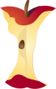Making Graphs That Represent DataPrintable Worksheets And Lessons

• Step-by-Step Lesson- Make a bar graph of the data chart and answer the questions that follow.

• Guided Lesson - This is three pages of problems. Each problem just has a single problem. I gave you plenty of work space.

• Guided Lesson Explanation - I hope I did a good job with these guys. It took me a good bit of time.

• Practice Worksheet - We just answer a series of questions from ten different data charts.

• Matching Worksheet - Match the bar graphs to the word problems in each case.(Click Here to Upgrade)

Homework Sheets

You are given data and then asked to create a bar graph and answer questions.

Practice Worksheets

Use the graph to understand the answers to the questions.

Math Skill Quizzes

At this level they focus on bar graphs. I will also introduce a line graph section that is geared for this level soon.

 Quiz 1 Quiz 2 Quiz 3